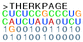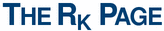## Documentation

The RK correlation coefficient can be computed from either computed KxK confusion matrices or two tables of NxK data points. The former, the discrete version, is computed using the script rkorrC and the latter by the script rkorr. In both cases it is assumed that wK = 1/K.

Compute RK from confusion matrices
On this webserver KxK confusion matrices on the form

C_11 C_12 ... C_1K C_21 C_22 ... C_2K ... C_K1 C_K2 ... C_KK
line by line can be computed. K can differ line by line, but it is recommended that K is the same. An output example is:
 R_K K b b' COV_XY COV_XX COV_YY Q_K C_11 C_12 C_13 C_21 C_22 C_23 C_31 C_32 C_33 0.75 3 0.76 0.75 1613192 2127864 2145578 0.85 213 21 12 89 459 90 29 39 958 0.79 3 0.78 0.79 5637032 7202094 7156148 0.85 984 58 23 239 929 59 95 12 894 0.05 3 0.05 0.05 3935755 83486406 83417218 0.37 34 3443 96 3467 89 32 13 49 3977
where R_K is the correlation coefficient, K the number of categories, b and b' the coefficients in the linear fits between the two data sets, and COV(X,Y), COV(X,X), COV(Y,Y) the respective expected covariances. Q_K is the normalized trace of the confusion matrix, and C_11, ..., C_33 are the elements of the confusion matrix. This was computed for the confusion matrix data example.

This option invoke the script rkorrC. [The commandline version has an option more, convenient when the script is applied in a pipe of data.]

Warning: use of different K's will imply that the header with C_ij unrealiable. The results line by line is still valid.

Compute RK from two NxK tables of data
Here, only one single R_K can be computed at the time. The input to the webserver should be data line by line of the form (n=1,...,N)

Y_n1, Y_n2, ... , Y_nK X_n1, X_n2, ... , X_nK
An output example
 R_K b b' COV(X,Y) COV(X,X) COV(Y,Y) 0.009105 0.102038 0.000813 8.267932 81.027805 10175.492523
where R_K is the correlation coefficient b and b' the coefficients in the linear fits between Y and X (and X and Y resp.), and COV(X,Y), COV(X,X), COV(Y,Y) the respective expected covariances. This was computed for the NxK table data example.

This option invoke the script rkorr.